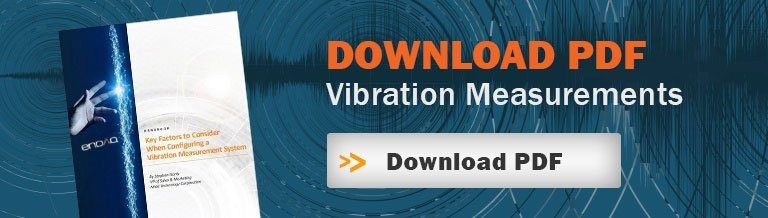Acquiring vibration data is only part of the challenge of vibration measurement; the other part is the analysis of the data acquired. It’s important to understand the types of waveforms associated with vibration analysis, the important differences between them and when it is appropriate to use each type of vibration analysis tool. Here’s a quick overview of some of the basics.

This blog will discuss and compare several different ways to analyze your vibration data. And for a deeper dive, read our handbook on Vibration Measurements.

Time Domain Vibration Analysis

Vibration analysis starts with a time-varying, real-world signal from a transducer or sensor. Analyzing vibration data in the time domain (amplitude plotted against time) is limited to a few parameters in quantifying the strength of a vibration profile: amplitude, peak-to-peak value, and RMS, which are identified in this simple sine wave.• The peak or amplitude is valuable for shock events, but it doesn’t take into account the time duration and thus the energy in the event.
• The same is true for peak-to-peak value with the added benefit of providing the maximum excursion of the wave, which is useful when looking at displacement information, specifically clearances.
• The RMS (root mean square) value is generally the most useful because it is directly related to the energy content of the vibration profile and thus the destructive capability of the vibration. RMS also takes into account the time history of the wave form.

Vibration is an oscillating motion about equilibrium so most vibration analysis looks to determine the rate of that oscillation or the frequency, which is proportional to the system’s stiffness. The number of times a complete motion cycle occurs during a period of one second is the vibration’s frequency and is measured in hertz (Hz). For simple sine waves, the vibration frequency could be determined from looking at the waveform in the time domain; however, as different frequency components and noise are added, spectrum analysis is necessary to obtain a clearer picture of the vibration frequency.

Frequency Domain Vibration AnalysisFast Fourier Transform (FFT)

The fast Fourier transform (FFT) is an efficient algorithm used to compute a discrete Fourier transform (DFT). This Fourier transform outputs vibration amplitude as a function of frequency so that the analyzer can understand what is causing the vibration. The frequency resolution in an FFT is directly proportional to the signal length and sample rate. To improve the resolution, the time of the recording must be extended, but be careful of a changing vibration environment.

Spectrogram

A spectrogram takes a series of FFTs and overlaps them to illustrate how the spectrum (frequency domain) changes with time. If vibration analysis is being done on a changing environment, a spectrogram can be a powerful tool to illustrate exactly how that spectrum of the vibration changes.

Power Spectral Density

A power spectral density (PSD) takes the amplitude of the FFT, multiplies it by its complex conjugate and normalizes it to the frequency bin width. This allows for accurate comparison of random vibration signals that have different signal lengths. For this reason, PSDs are typically used to describe random vibration environments like those specified in military and commercial test standards.

Summary

To learn more about vibration analysis, read my blog “Vibration Analysis: FFT, PSD, and Spectrogram Basics.”  Also check out our handbook on Vibration Measurements. Or for guidance on the most appropriate approach to vibration analysis for your application, contact us for guidance.hbspt.cta._relativeUrls=true;hbspt.cta.load(637862, '73532778-1400-461a-ad39-2aa3396db856', {});Posted by:
Steve Hanly on October 11, 2018
Find me on: